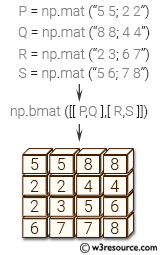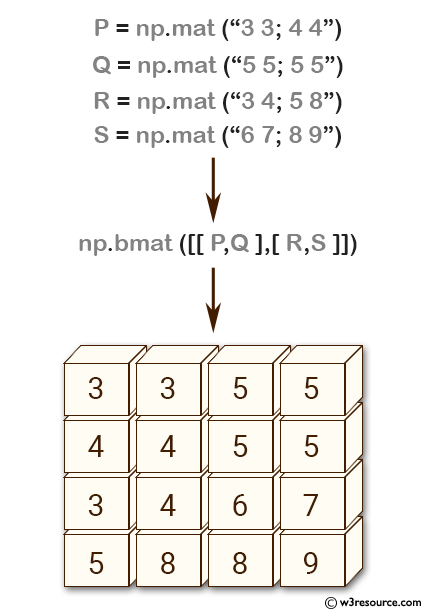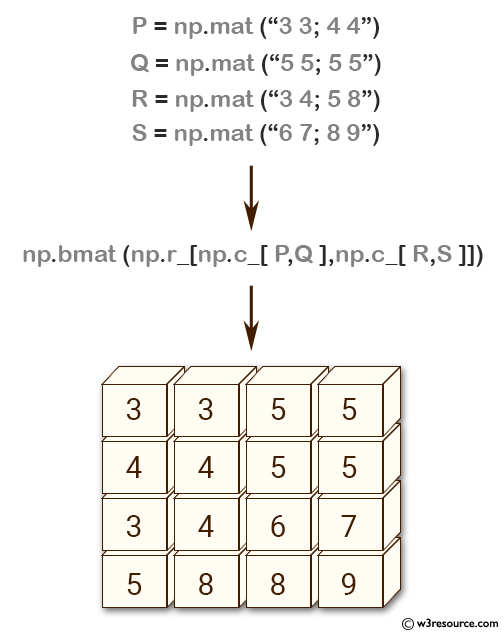# NumPy array creation: bmat() function

## numpy.bmat() function

The numpy.bmat() function is used to built a matrix object from a string, nested sequence, or array.

Syntax:

`numpy.bmat(obj, ldict=None, gdict=None)`Version: 1.15.0

Name Discription Required / Optional
obj Input data. If a string, variables in the current scope may be referenced by name. Required
ldict A dictionary that replaces local operands in current frame. Ignored if obj is not a string or gdict is None. Optional
gdict A dictionary that replaces global operands in current frame. Ignored if obj is not a string. Optional

Return value:

matrix - Returns a matrix object, which is a specialized 2-D array.

All the following expressions construct the same block matrix:

Example-1: NumPy.bmat() method

``````>>> import numpy as np
>>> P = np.mat('3 3; 4 4')
>>> Q = np.mat('5 5; 5 5')
>>> R = np.mat('3 4; 5 8')
>>> S = np.mat('6 7; 8 9')
>>> np.bmat([[P,Q], [R, S]])
matrix([[3, 3, 5, 5],
[4, 4, 5, 5],
[3, 4, 6, 7],
[5, 8, 8, 9]])
``````

Pictorial Presentation:Example-2: NumPy.bmat() method

``````>>> import numpy as np
>>> P = np.mat('3 3; 4 4')
>>> Q = np.mat('5 5; 5 5')
>>> R = np.mat('3 4; 5 8')
>>> S = np.mat('6 7; 8 9')
>>> np.bmat(np.r_[np.c_[P, Q], np.c_[R, S]])
matrix([[3, 3, 5, 5],
[4, 4, 5, 5],
[3, 4, 6, 7],
[5, 8, 8, 9]])
>>> np.bmat('P, Q; R, S')
matrix([[3, 3, 5, 5],
[4, 4, 5, 5],
[3, 4, 6, 7],
[5, 8, 8, 9]])
``````

Pictorial Presentation:Python - NumPy Code Editor:

Previous: mat()
Next: NumPy Array manipulation Home

﻿